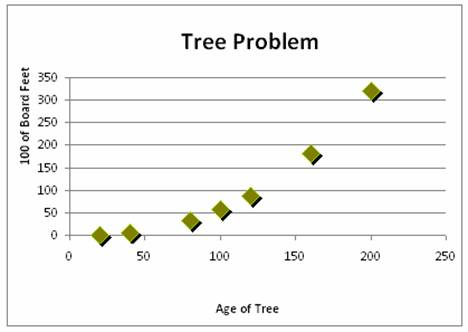# 2620 Scatter Plot Transformations The Circle

20 Questions | Attempts: 253
ShareSettings• 1.
In Quadrant 1, what transformations are applicable:
• A.

X squared

• B.

Y squared

• C.

Reciprocal x

• D.

Reciprocal y

• E.

Log x

• F.

Log y

• 2.
In Quadrant 2, what transformations are applicable:
• A.

X squared

• B.

Y squared

• C.

Reciprocal x

• D.

Reciprocal y

• E.

Log x

• F.

Log y

• 3.
In Quadrant 3, what transformations are applicable:
• A.

X squared

• B.

Y squared

• C.

Reciprocal x

• D.

Reciprocal y

• E.

Log x

• F.

Log y

• 4.
In Quadrant 4, what transformations are applicable:
• A.

X squared

• B.

Y squared

• C.

Reciprocal x

• D.

Reciprocal y

• E.

Log x

• F.

Log y

• 5.
Which Quadrants are appropriate for an x squared transformation
• A.

• B.

• C.

• D.

• 6.
Which Quadrants are appropriate for a y squared transformation
• A.

• B.

• C.

• D.

• 7.
Which Quadrants are appropriate for a log x transformation
• A.

• B.

• C.

• D.

• 8.
Which Quadrants are appropriate for a log y transformation
• A.

• B.

• C.

• D.

• 9.
Which Quadrants are appropriate for a 1/y transformation
• A.

• B.

• C.

• D.

• 10.
Which Quadrants are appropriate for a 1/x transformation
• A.

• B.

• C.

• D.

• 11.
What is the effect of transformations in Quadrant 1
• A.

They stretch x values

• B.

They stretch y values

• C.

They compress x values

• D.

They compress y values

• 12.
What is the effect of transformations in Quadrant 2
• A.

They stretch x values

• B.

They stretch y values

• C.

They compress x values

• D.

They compress y values

• 13.
What is the effect of transformations in Quadrant 3
• A.

They stretch x values

• B.

They stretch y values

• C.

They compress x values

• D.

They compress y values

• 14.
What is the effect of transformations in Quadrant 4
• A.

They stretch x values

• B.

They stretch y values

• C.

They compress x values

• D.

They compress y values

• 15.
Which quadrant is an appropriate shape for the scatter plot above?
• A.

• B.

• C.

• D.

• E.

None of these

• 16.
Which quadrant is an appropriate shape for the scatter plot above?
• A.

• B.

• C.

• D.

• E.

None of these

• 17.
Which quadrant is an appropriate shape for the scatter plot above?
• A.

• B.

• C.

• D.

• E.

None of these

• 18.
Which quadrant is an appropriate shape for the scatter plot above?
• A.

• B.

• C.

• D.

• E.

None of these

• 19.
Which quadrant is an appropriate shape for the scatter plot above?
• A.

• B.

• C.

• D.

• E.

None of these

• 20.
Which quadrant is an appropriate shape for the scatter plot above?
• A.

• B.

• C.

• D.Back to top